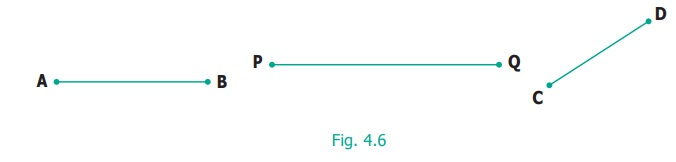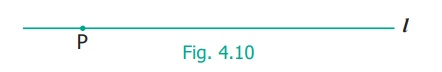Home | | Maths 6th Std | Line segment

# Line segment

What do we call a line that is short and ends on both sides?

Line segment

What do we call a line that is short and ends on both sides? That we call it as a line segment and name both of its ends with letters as shown in fig. 4.6.We usually use CAPITAL LETTERS to denote the ends of the line segments. A line segment is denoted by. What can we do with a line segment? We can measure its length. Given two line segments, we can compare their lengths and say which is shorter and which is longer.

Even if we measure length as a number, we get lots of line segments, each with a definite length. Using a ruler, we can draw the following line segments.

1 cm A ____ B

2 cm A ______ B

3 cm A ____________ B

...

10 cm A ________________________ B

What about a line segment of length 17 cm or 20 cm or 30 cm or 378 cm? Like, numbers never end, line segments get longer and longer forever!

Try this

Name all the line segments.EA, EB, ED, EC, AB, CD

1. Construction of line segment

Learn to measure line segments using the ruler.

Correct way of viewing the scale.Examples of Measuring Line SegmentsTry this

If AB = 5cm, say which of the measures are correct in fig. 4.9.Geometry originated from two Greek words, geo, meaning earth and metron meaning measure. Geometry means earth measure. Around 600 B.C., Thales of Miletus was the first to use deductive methods to develop geometric concepts. The Greek Mathematician, Pythagoras continued the systematic development of Geometry.

Example

With the help of a ruler and compass, draw a line segment= 5.5cm.

Solution

Draw a line ‘ l ’ and mark a point ‘P’ on it as shown in Fig. 4.10.Measure 5.5 cm using compass as shown in Fig. 4.11 placing the pointer at ‘0’ and the pencil pointer at 5.5 cm.Place the pointer of the compass at ‘ P ’ then draw a small arc on the line ‘l ’ with the pencil pointer (Fig. 4.12). It cuts the line ‘ l ’ at a point and name that point as ‘Q’ (Fig. 4.13).Now,is the required line segment of length 5.5 cm.

Activity

This game can be played in small groups. Take 10 or more sticks of equal length. Join them as a bundle and put them on the floor in such a way that they fall one above the other. The challenge is to take one stick after other without disturbing the position of the other sticks.Activity

Enjoy trying kolams using line segments!Tags : Geometry | Term 1 Chapter 4 | 6th Maths , 6th Maths : Term 1 Unit 4 : Geometry
Study Material, Lecturing Notes, Assignment, Reference, Wiki description explanation, brief detail
6th Maths : Term 1 Unit 4 : Geometry : Line segment | Geometry | Term 1 Chapter 4 | 6th Maths